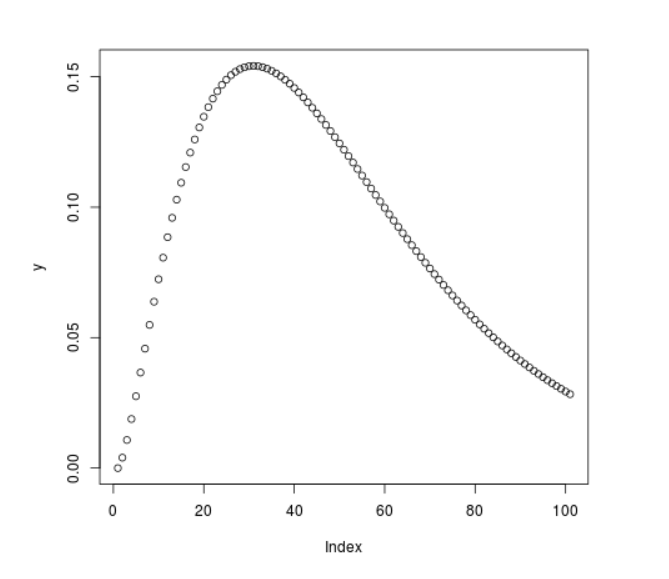# Compute Chi Square Density in R Programming – dchisq() Function

`dchisq()` function in R Language is used to compute chi square density for a vector of elements. It also creates a density plot for chi square distribution.

Syntax: dchisq(vec, df)

Parameters:
vec: Vector of x-values
df: Degree of Freedom

Example 1:

 `# R program to compute  ` `# Chi Square Density ` ` `  `# Create a vector of x-values ` `x <``-` `seq(``0``, ``10``, by ``=` `1``) ` ` `  `# Calling dchisq() Function ` `y <``-` `dchisq(x, df ``=` `5``) ` `y `

Output:

```  0.00000000 0.08065691 0.13836917 0.15418033 0.14397591 0.12204152
 0.09730435 0.07437127 0.05511196 0.03988664 0.02833456
```

Example 2:

 `# R program to compute  ` `# Chi Square Density ` ` `  `# Create a vector of x-values ` `x <``-` `seq(``0``, ``10``, by ``=` `0.1``) ` ` `  `# Calling dchisq() Function ` `y <``-` `dchisq(x, df ``=` `5``) ` ` `  `# Plot a graph  ` `plot(y) `

Output:My Personal Notes arrow_drop_upCheck out this Author's contributed articles.

If you like GeeksforGeeks and would like to contribute, you can also write an article using contribute.geeksforgeeks.org or mail your article to contribute@geeksforgeeks.org. See your article appearing on the GeeksforGeeks main page and help other Geeks.

Please Improve this article if you find anything incorrect by clicking on the "Improve Article" button below.

Article Tags :

Be the First to upvote.

Please write to us at contribute@geeksforgeeks.org to report any issue with the above content.